E-mail
New member
New customer
Discover our services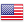Settings
Dynamic quotes
OFF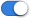ON
 Investment themes Smart City, investing in a megatrend
Investment themes
The path of modernity opens the way to a more connected, safer and more ecological city. The smart city aims to improve the quality of life of city dwellers by making urban spaces more adaptive and efficient on a daily basis.

To achieve this, urban development relies on an ecosystem of intelligent objects and services thanks to new technologies. This management method covers all aspects of a city, starting with public and private infrastructures (buildings, street furniture, home automation), networks (water, gas, electricity, telecoms, waste management), transport (soft mobility, public transport, roads, intelligent cars) and e-services.

The smart city market has been growing rapidly for several years. While the rural exodus is accelerating, urbanization is in full swing. According to UN estimates, two out of three people will be living in cities by 2030. The need to reorganize urban spaces is closely linked to the migration, climate and technological challenges of our century.

This thematic list identifies the main actors of tomorrow's smart cities. Regularly updated, this list includes about 100 companies with sufficient capitalization and liquidity and solid fundamentals.To access our selection of stocks, regularly updated with new opportunities, discover these tailor-made subscriptions featuring our exclusive tools >> Discover
 Sector Capi. (M\$) 1st Jan %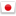░░░░░ ░░░░░░░░░░░ ░░░░░ ░░░░░░░░░░ ░░░░░░░░░ ░ ░░░░░░░░░ ░ ░░░ ░░.░░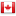░░░░░░ ░░░░░░░░░░░ ░░░░░ ░░░░░░ ░ ░░░░ ░░░░░░░░ ░ ░░░ ░░.░░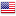░░░░░ ░░░░░░░░░░░ ░░░░░ ░░░░░ ░░ ░░░ ░░░.░░░░░░░░░░░░░ ░░░░ ░░░░░░░░░░ ░ ░░░ ░░.░░░░░░░ ░░░░░░░░░░░ ░░░░░ ░░░░░░░░░░░░░░ ░ ░░░░░░░░░░ ░ ░░░ ░░.░░░░ ░░░░░░░░░░░░░ ░░░░░░░░░░░ ░░░░░ ░░░░░░░░░░░░░░ ░░ ░░░ ░░.░░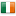░░░░░░░░ ░░░░░ ░░░ ░░░░░░░░ ░ ░░░░░░░░ ░░░░ ░░░░░░░░░░░░░ ░░ ░░░ ░░.░░░░░░░░░░ ░░░░░░░ ░░░░░░░░ ░░░░░░░░ ░ ░░░░░░░░░░░░ ░░░░░░░░ ░ ░░░ ░░.░░░░░░ ░░░░░░ ░░░░░░ ░░░ ░░░░ ░░░░░░░ ░ ░░░░░░░░ ░ ░░░ ░░.░░░░░░░░░░░ ░░░░ ░░░░░ ░░░░░░░░░░░░░░ ░░░ ░░░ ░░.░░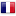░░░░░░░░░░░░░░░░░░ ░░░░ ░░░░░ ░░░░░░░░░░░░░░ ░░ ░░░ ░░.░░░░░░░░░░░ ░░░░░░░░░░░ ░░░░░ ░░░░░░░░ ░ ░░░ ░░░ ░░.░░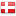░░░░░░░░ ░░░░░░░░░░░░░ ░░░ ░░░░░░░░░░░░ ░░░░░░░░ ░ ░░░ ░░.░░░░░░░░░ ░░░ ░░░░░ ░░░░░░░░░░ ░░░░░░░░░ ░ ░░░░░░░░░ ░░ ░░░ ░░.░░░░░░░░░░ ░░░░░░░░░░░ ░░░░░░░ ░ ░░░░░░░░ ░ ░░░ ░░.░░░░░░░░░░░ ░░░░ ░░░░░░░░░░░░░ ░░░░░░░ ░░░░░░░░░ ░ ░░░░░░░ ░░ ░░░ ░░.░░░░░░░░░░░░ ░░░░░░░░░░░ ░░░░ ░░░░░░░░░░ ░░░░░░░░░░ ░░░░░░░░ ░ ░░░ ░░░.░░░░░░░░░░░ ░░░░ ░░░░░ ░░ ░░░░░░░░ ░ ░░░░░░░░░░ ░░ ░░░ ░░░.░░░░░░░░ ░░░░░░░░░░░ ░░░░░ ░░░░░░░░░░░░░░ ░░░ ░░░ ░░░.░░░░░░░░░ ░░░░░░░░░░░ ░░░░░ ░░░░░░░░░░ ░░░░░░░░░░ ░ ░░░░░░░░░ ░░░ ░░░ ░░.░░░░░░░░░░ ░░░░░ ░░░░░░░░ ░░░░░░░░░░░ ░ ░░░ ░░.░░░░░░░░ ░░░░░░░░░ ░░░░ ░░░░░░░░ ░░░░░░░░ ░ ░░░░░░░░░░░░ ░░░░░░░░ ░░░ ░░░ -░░.░░░░░░░░░░░ ░░░░░░░ ░░░░░░░░░░░ ░░░░░░░░░░ ░░░░░░░░ ░ ░░░ -░░.░░░░░░░░░ ░░░░░░░ ░░░░ ░░░░ ░░░░░ ░░░░░░░░░░░░ ░ ░░░░░░░░░░░ ░ ░░░ ░░.░░░░░ ░░░░░░░░░░░ ░░░░░ ░░░░░░░░░░ ░░░░░░░░░ ░ ░░░░░░░░░ ░ ░░░ ░.░░░░░░░░ ░░ ░░░░░ ░░ ░░░░░░░░ ░ ░░░░░░░░░░ ░ ░░░ ░░.░░░░░░░░░░░ ░░░░░ ░░░░░░░░░░░ ░░░░ ░░░░░ ░░░░░ ░░░░░ ░ ░░░░░░░░░ ░ ░░░ ░░.░░░░░░░ ░░░░░░░░ ░░░░ ░░░░░ ░░░░░░░░░░░░░ ░░░░░░░░ ░ ░░░░░░░░░ ░ ░░░ ░░.░░░░░░░░░░ ░░░░░ ░░░░░░░░░░░░ ░ ░░░░░░░░░░░ ░░ ░░░ -░░.░░░░░░░░░ ░░░░ ░░░░░░░░░░ ░░░░░░░░ ░░ ░░░ ░░.░░░░░░░░░░ ░░░░░░░░ ░░░░ ░░░░ ░░░░ ░ ░░░░░░░ ░░░░░░░░░ ░ ░░░ ░░.░░░░░░░░░░░░░░░ ░░░░ ░░░░░ ░░░░░░░░░░░░░░ ░ ░░░░░░░░░░ ░ ░░░ ░░.░░░░░░░░░░░░ ░░░░░░░░░░░░░░░░░░ ░░░░░░░ ░░░░░░░░ ░░░░░░░ ░ ░░░ -░░.░░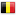░░░░░░░ ░░░░ ░░░░░ ░░░░░░░░░░░░░░ ░ ░░░ ░░.░░░░░░░ ░░░░░░░░ ░░░░ ░░░░░ ░░░░░░░░░░░░░░ ░ ░░░░░░░░░░ ░░░ ░░░ ░░.░░░░░ ░░░░ ░░░░░░░░░░░ ░░░░░░░░ ░░ ░░░ -░.░░░░░░░░░ ░░░░ ░░░░░ ░░░░░░░░░░ ░░░░░░░░░░ ░ ░░░░░░░░░ ░░ ░░░ ░░.░░░░░░░ ░░░░░░░░░░░░░ ░░░░ ░░░░░ ░░░░░░░░░░░░░░ ░ ░░░ ░░.░░░░░░░░░░ ░░░░░░░░░░░░░ ░░░░ ░░░░░░░░░░ ░░░░░░░░░ ░ ░░░░░░░ ░░░░░░░░░░░ ░░ ░░░ ░░.░░░░░░░ ░ ░░░░░░░ ░░░░░░░░░░░░ ░░░░░░░░░ ░░░ ░░░ ░░.░░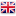░░░░░░░ ░░░░░░░░░░░░░░ ░░░ ░░░░░ ░░░░░░░░░░░░░░ ░ ░░░░░░░░░░ ░ ░░░ ░.░░░░░░░░░░░░░░ ░░░░░░░░░░░ ░░░ ░░░░░ ░░░░░░░░░░ ░░░░░░░░░ ░ ░░░░░░░░░ ░░ ░░░ ░░.░░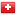░░░░░░░ ░░░░░░░ ░░ ░░░░░░░ ░ ░░░░░░░░░ ░░░░░░░░░ ░ ░░░ ░░.░░░░░░░░░░░░ ░░░░ ░░░░░ ░░░░░░░░░░░░░░ ░ ░░░ ░░░.░░░░░░░░░░░░ ░░░░░░░░ ░░░░░░░░ ░ ░░░░░░░░░░░░ ░░░░░░░░ ░░░ ░░░ -░░.░░░░░░░ ░░░░░ ░░ ░░░░░ ░░░░░░░░░░ ░░░░░░░░░ ░ ░░░░░░░░░ ░░ ░░░ ░░.░░░░░░░░░░ ░░░░░░░░░░ ░░░░ ░░░░░░░░░░ ░░░░░░░░ ░░ ░░░ -░.░░░░░░░░░░░░ ░░░░ ░░░░░ ░░░░░░░░ ░░░░░░░░ ░ ░░░ -░░.░░░░░ ░░░ ░░░░░ ░░░░░ ░░░░░░░░░░ ░░░░░░░░░ ░░ ░░░ ░░.░░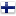░░░░░ ░░░ ░░░░░ ░░░░░░░░░░░░░░ ░ ░░░░░░░░░░ ░░ ░░░ ░░.░░░░░░░ ░░░░░░░░ ░░░ ░░░░░ ░░░░░░░░░░ ░░░░░░░░░░ ░ ░░░░░░░░░ ░ ░░░ ░░.░░░░░░░ ░░░░░░░░░░░ ░░░░░ ░░░░░░░░░░ ░░░░░░░░░░ ░ ░░░░░░░░░ ░ ░░░ -░.░░░░░░░░░ ░░░░░░░ ░░ ░░░░░░ ░░░░░░░░ ░░░░░░░░░░░ ░ ░░░ ░░.░░░░░░░░░░ ░░░░░░░░░░░░ ░░ ░░░░░ ░░░░░░░░░░░░░░ ░░ ░░░ ░░.░░░░░░░░░░░░░░░░ ░░░░ ░░░░░░░░░░░ ░░░░░░░░ ░░ ░░░ ░░.░░░░░ ░░░░░░░░░░░░ ░░░░ ░░░░░░░░░░░ ░░░░░ ░░ ░░░ ░░.░░░░░░░░ ░░░░░░░░░░░ ░░░░░ ░░░░ ░░░░░ ░░░░░░░░░░░░ ░ ░░░░░░░░░░░ ░░ ░░░ ░░.░░░░░░░░░ ░░░ ░░░░░ ░░ ░░░░░░░░ ░ ░░░░░░░░░░ ░ ░░░ ░░.░░░░░░░░░░░░░░░░░ ░░░░░░░░░░░ ░░░░░ ░░░░░░░░░░ ░░░░░░░░░ ░ ░░░░░░░░░ ░░ ░░░ ░░.░░░░░░░░ ░░░░░░░ ░░░░░ ░░ ░░░░░░░░ ░ ░░░░░░░░░░ ░ ░░░ -░.░░░░░ ░░░░░░░░░░░ ░░░░░░░ ░░░░░░░ ░░░░░░░░░ ░░ ░░░ ░░.░░░░░░░░░░░ ░░░░░░░░ ░░ ░░░░░ ░░░░░░░░░░ ░░░░░░░░░░ ░ ░░░░░░░░░ ░░░ ░░░ ░░.░░░░░░░░░ ░░░░░ ░░░░░░░░░░ ░░░░░░░░░░ ░ ░░░░░░░░░ ░░ ░░░ ░░.░░░░░░░ ░░░ ░░░░░ ░░░░░░░░░░ ░░░░░░░░░ ░ ░░░░░ ░░ ░░░ ░░.░░░░░░░░ ░░░░░░░░░░░░░ ░░░░ ░░░░░ ░░ ░░░░░░░░ ░ ░░░░░░░░░░ ░░ ░░░ ░.░░░░░░░░░ ░░░░░░ ░░░░ ░░░░░░░░ ░░░░░ ░ ░░░ ░░.░░░░░░░░░░ ░░░░░░░ ░░░░░░░░░░ ░░░░░░░░░ ░ ░░░░░░░░░ ░░░░░░░░░ ░░ ░░░ ░░.░░░░░░░░░ ░░ ░░░░░░░░ ░░░░░░░░ ░ ░░░░░░░░ ░░ ░░░ ░░.░░░░░░░ ░░░░ ░░░░░ ░░░░░░░░ ░░░░░░░░ ░░ ░░░ -░░.░░░░░░░░░░░ ░░░░░░░ ░░ ░░░░░░░░ ░ ░░░░░░░░░ ░░░░░░░░░ ░░ ░░░ ░.░░░░░░░ ░░░ ░░░░░ ░░░░░░░░░░░ ░░░░░░░░ ░ ░░░░░░░░░ ░░░░░░░░ ░ ░░░ ░░.░░░░░░░░ ░░░░░░░░ ░░░░ ░░░░░░░░░░ ░░░░░░░░░ ░░░░░░ ░░ ░░░ ░░.░░░░░░░░░░ ░░░ ░░░░░ ░░░░░░░░░░ ░░░░░░░░░ ░ ░░░░░ ░ ░░░ ░░.░░░░░░░░░░░░ ░░░░░ ░░░░░░░░ ░░░░ ░░░░░ ░░░░░░░░░░░░░░ ░░ ░░░ ░░.░░░░░░░░ ░░░░░░ ░░░░ ░░░░░░░ ░ ░░░░░░░░░ ░░░░░░░░░ ░ ░░░ ░.░░░░░░░░░░░░ ░░░░░ ░░░ ░░░░░ ░░ ░░░░░░░░ ░ ░░░░░░░░░░ ░ ░░░ ░.░░░░░░ ░░░░░░░░░░░ ░░░░ ░ ░░░░░░░ ░░░░░░░░░ ░░ ░░░ ░░.░░░░░░░░░ ░░░░░░░░░░░░ ░░░░░░░ ░░░ ░░░░░ ░░░░░░░░░░ ░░░░░░░░░ ░ ░░░░░ ░ ░░░ ░.░░░░░░░░ ░░░░ ░░░░░ ░░░░░░░░░░░ ░░░░░░░░ ░ ░░░░░░░░░ ░░░░░░░░ ░ ░░░ ░░.░░░░░░░░░░░ ░░░░ ░░░░░░░░░░░ ░░░░░░░░ ░░ ░░░ -░░.░░░░░░░░░░ ░░░░░ ░░░░░░░░░░░░░░ ░ ░░░░░░░░░░ ░░ ░░░ -░.░░░░░░░░░░░░░ ░░░░░░░░░ ░░░░░░░░░░░░ ░░░░ ░░░░░░░░░░░ ░░░░░ ░░ ░░░ ░░.░░░░░░░ ░░░░░░░░░░░ ░░░░░░░░ ░░░ ░░░░░ ░░░░░░░░░ ░ ░░░░░░░ ░ ░░░ ░░.░░░░░░░░░░ ░░░░░░░░░░ ░░░░ ░░░░░ ░░░░░░░░░░░░░░ ░ ░░░░░░░░░░ ░░ ░░░ ░░.░░░░░░░░░ ░░░░░░░░░░░░░░ ░░░░░ ░░░░░░░░░░ ░░░░░░░░░░░░░░░░░░ ░░░░░░░░ ░░░ ░░░ -░░.░░░░░░░ ░░░░░░ ░░░░░░░░░░░ ░░░░░ ░░░░░░░ ░░░ ░░░░░░░░░ ░░ ░░░ -░.░░░░░░░░░ ░░░░░░░░░ ░░░░ ░░░░░░░ ░░░░░░░░░ ░░ ░░░ ░░.░░░░░░░░ ░░░░░░░░░░░░░ ░░░░░ ░░░░░ ░░░░░░░░░ ░░ ░░░ ░░.░░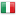░░░░░ ░░░░░░ ░░░░░░░░░░░░░░░░░░ ░░░░░░░ ░░░░░░░░░░░░░░ ░░ ░░░ ░.░░
 Heatmap % Price Change by Sector 1 day 5 days 1st jan. #heatmap_liste_thema .highcharts-tooltip>span { background-image: linear-gradient(#FFFFFF,#EEEEEE); border-radius: 5px; padding: 8px; z-index: 10; } .highcharts-tooltip>span a { color: #004EFF !important; } Sector allocation Ratings chart - Surperformance Ratings ESG Refinitiv